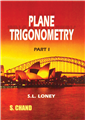Home > Higher Education > Mathematics > Plane Trigonometry, Part-I# Plane Trigonometry, Part-I

## S L Loney

 ISBN : 9788121908016 Pages : 350 Binding : Paperback Language : English Imprint : S. Chand Publishing Trim size : 4.75" X 6.75" inches Weight : 0.5kg
 List Price :150.00
 Offer Price :120.00

20% OFF

# In Stock

Credit Card / Debit Card / Internet BankingMeasurement of Angles; Sexagesimal, Centesimal and Circular Measure, Trigonometrical Ratios for Angles less than a Right Angle  Simple Problems in Heights and Distances,  Application of Algebraic Signs to Trigonometry,  Trigonometrical Functions of Angles of any Size and Sign,  General Expression for all Angles Having a Given Trigonometrical Ratio,  Trigonometrical Ratio of the Sum and Difference of Two Angles,  The Trigonometrical Ratio of Multiple and Submultiple Angles,  Identities and Trigonometrical Equations,  Logarithms,  Tables of Logarithms and Trigonometrical Ratios, Principle of Proportional Parts,  Relations Between the Sides and the Trigonometrical Ratios of the Angles of any Triangle,  Solution of Triangles,  Heights and Distances,  Properties of a Triangles,  On Quadrilaterals and Regular Polygons, 17. Trigonometrical Ratios of Small Angles, Area of a Circle, DIP of the Horizon  Inverse Circular Functions,  On Some  Simple Trigonometrical Series,  Elimination,  Projections • Miscellaneous Examples • Answers • Table of Logarithms, Natural Sines, Cosines and Tangents, Logarithms Sines, Cosines Tangents and Radian Measure of Angles### More Books By Author#### Plane Trigonometry Part II ...

978812190918199.0079.20

20% OFF150.00120.00